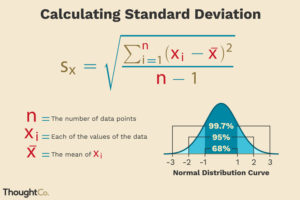# Java Standard Deviation in 4 Easy Ways | Java Programs

Java code To Calculate Standard Deviation – In this article, we will brief in on all the possible ways to calculate standard deviation in Java Programming. Suitable examples and sample programs have been included in order to make you understand simply. The compiler has also been added so that you can execute the programs yourself.

• Using Standard Method
• Using Scanner Class
• Using Command Line Arguments
• Using Static Method
• Using Separate Class

Standard Deviation in general terms can be explained as the divergence of the participants from the mean value among the group of values.

Mathematically, it is the same. It is the quantity which expresses the variation of the group from the mean value.Image Source : ThoughtCo

As you can see, the formula of Standard Deviation is as follows:

S = [(Sum of (xix)2)/n-1]^1/2

where

• n = number of data points
• xi = values of the data
• x = Mean

Thus, the various ways to calculate the standard deviation in Java Programming is as follows:

## Standard Deviation Java Code

This methodology is where the entire code is written in the main method itself.

Here, the input is an array of integers or decimal numbers hence we take the input type to be double.

Using double type, after calculations performed on this, our end result will also be a double. So our result Standard Deviation is of double type as well.

In the standard method we take inputs in the code itself which are fixed and the length of array is also fixed. In order to arrive at the standard deviation of the set of numbers we have step wise process.

sum=sum+input[i];

• After this the mean has to be found. The formula for mean is: Mean=Sum/Total Number.

mean=sum/n;

sum+=Math.pow((input[i]-mean),2);      mean=sum/(n-1);

• Lastly, Standard Deviation is square-root of variance.

deviation=Math.sqrt(mean);

Output:

Using Scanner Class

Any value predetermined decreases the efficiency of code. In such a scenario, if something as important as input is fixed then the code is not at its optimal state.

Using Scanner Class in Java, inputs can be read at runtime and can be given according to the user/tester and can always be changed without the strain of going through the code.

So at run time itself when the input is being read using scanner class, the sum is being performed. Followed by which we find the mean, variance and standard deviation as mentioned above.

Output:

### Using Command Line Arguments

Another way of taking input from the user is via command line arguments. When giving the run command, the number of elements followed by the elements can be given in the command itself with a space in between each of them.

These arguments can then be stored in the variables such that arg is the number of elements (n) or size of array followed by n elements as individual elements of the array.

While storing these values, they are converted into integers or long type based on requirement. After gathering these values the same steps as mentioned above are to be followed.

#### Using Static method

In all the above methods, the entire code was written in the main method only.

Doing that could give rise to difficulties when someone wants to make changes.

So, to overcome this we can make use of a separate static method within the same class.

The gathering of essential inputs can be performed in the main method and then this separate static method (Calculations) can be called in the main method.

This static method (Calculations) can then contain all the necessary operations that are to be performed by finding mean and finally the standard deviation.

Output1:

x

## Plus Star Pattern Java Program | Patterns

Java program to print plus star pattern program – We have written the below print/draw ...